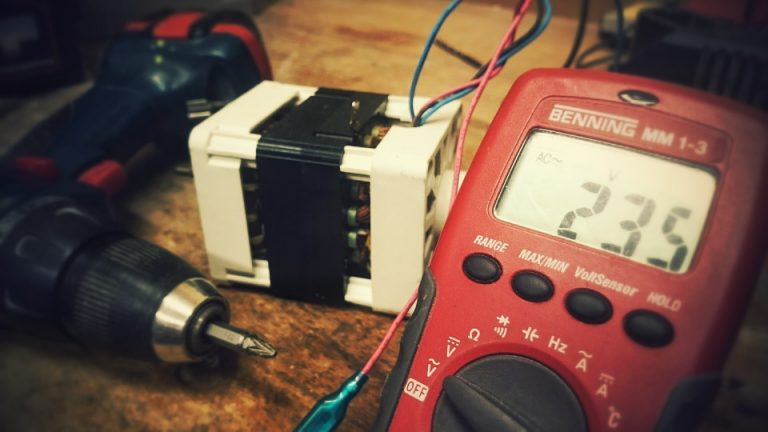#### \$45 Off Today *Conditions apply#### Call Us# What is the Difference Between a Watt and an Amp

When talking about electricity, power, and electrical appliances, it is most likely that you will come across such words like watts and amps. Although these words are commonly used, most people may not have any idea regarding the difference between the watt and an amp. This article will try to explain what is the difference between a watt and an amp. Knowing the difference between the two words can be of benefit to any homeowner because this knowledge would allow them to be potentially aware of how to save on electricity bills.

An amp is the measure of the amount of current or electrical energy that is passing through an electric line whereas a watt is the unit for measuring electrical power, and it is calculated by multiplying the voltage (volts) of a particular source of electricity and the current (amps) being used by an appliance. Essentially, electric power is the product of current and voltage.

One similarity between a watt and an amp is that they can both be measured using special devices or meters designed for this purpose. An ammeter is the device used to measure the current being used by an electrical device in amps. For the power in the electrical system, one can purchase a wattmeter to measure the same directly. Additionally, the power in the electrical system can be calculated by measuring the current in amps and then multiplying this value by the voltage. In most cases, different places have a standard for the voltage, and this means that one would only need to measure the current in amps and then check the voltage standard for their locality and multiply the two. For example, if an electrical device uses 5 amps and the voltage is 36 volts, the power would be (5*36) = 180 Watts.

As you may have noticed, electricity bills are indicated in terms of kilowatts, and in this case, a kilowatt is the short form for 1000 watts. Since a watt is the product of voltage and current, if you increase the amps, the watts will also increase. What this means is that using appliances that use less amps will reduce the power or watts used and consequently the electricity bills.

For more information, anyone can be assisted by CLF Services, a company that offers professional electrician services to residential, industrial, and commercial customers in Brisbane, Australia.